### Geometry Nodes

The geometry nodes are the leaf nodes of the scene hiearchy which actually contain geometry. The basic object, as defined by the object statement, is a collection of polygons. The other primitive objects defined in SLIDE are the parameterized surfaces: sphere, cylinder, cone, torus, cyclide, bsplinecurve, bezeircurve, polyline, sweep, bsplinepatch, and bezeirpatch. The object differs from these other surface primitives because it is not defined over a rectangular domain in texture space. The object does not have a field for adjusting this texture parameterization, and instead the texture coordinates for the faces which comprise an object are defined within the point entity.

All geometric nodes may have these fields:

SLIDE Definition tclinit Definition
 primitive_object id texture ( texture_triple ) [( texture_triple )] ( texture_triple ) [( texture_triple )] solid solid_flag surface surface_id shading shading_flag lod lod_flag ribbegin "rib_string" ribend "rib_string" endprimitive_object
 slide create primitive_object id \ -texture { texture_triple } [{ texture_triple }] { texture_triple } [{ texture_triple }] \ -solid solid_flag \ -surface surface_id \ -shading shading_flag \ -lod lod_flag \ -ribbegin "rib_string" \ -ribend "rib_string" \

SLIDE Defaults tclinit Defaults
 primitive_object id texture ( `0.0` `0.0` ) [( `1.0` `0.0` )] ( `1.0` `1.0` ) [( `0.0` `1.0` )] solid `SLF_SOLID` surface `SLF_INHERIT` shading `SLF_INHERIT` lod `SLF_FULL` ribbegin `SLF_NULL` ribend `SLF_NULL` endprimitive_object
 slide create primitive_object id \ -texture `{ 0.0 0.0 }` [`{ 1.0 0.0 }`] `{ 1.0 1.0 }` [`{ 0.0 1.0 }`] \ -solid `\$SLF_SOLID` \ -surface `\$SLF_INHERIT` \ -shading `\$SLF_INHERIT` \ -lod `\$SLF_FULL` \ -ribbegin `\$SLF_NULL` \ -ribend `\$SLF_NULL`

Field Description
texture

Two or four (u, v, w) triples of texture coordinates, A, C or A, B, C, D respectively. These values are in texture space and define how to map the texture onto the geometry of the surface. A, B, C, D run around the contour of the surface patch in counterclockwise order. If only A, C are specified then B = (Cu, Av) and D = (Au, Bv). This type of modification to the texture parameterization is convenient for tiling a texture over a surface.

Complete Texture on a Square Texture Tiled 4X on a Square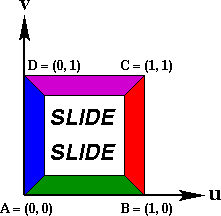The four texture coordinate points A, B, C, D can be used to do the same tiling operation in a less convenient way or to crop nonrectangular quadrilaterals out of the texture image. An example of this would be clipping out a triangular region from a texture image to map on a degenerate surface patch. If the complete texture image is used on a degenerate quadrilateral patch then the texture will take on unpredictable warping behaviors.

Triangular Texture Region
Complete Texture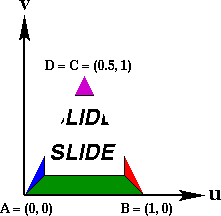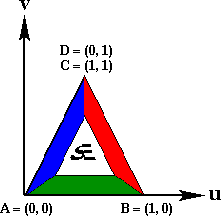The abstraction described above assumes a rectangular texture domain with a right handed coordinate system. The reality is that most bitmap image formats, including GIF, are defined with a left handed coordinate system, the y-axis pointing down. To make the abstraction valid, it is useful to store texture map images mirrored in the y-direction.

Texture Domain Abstraction Reality of GIF and
other Image FormatsNOTE: This field does not apply for object.

solid See the Solid Type
lod See the LOD Type

Example: primitives.slf

The SLIDE file primitives.slf is an interactive demo of all of the SLIDE geometric primitive nodes. This file allows the viewer to select a primitive to work with. When a new primitive is selected GUI for that type of primitive appears, so that the viewer can interactive adjust parameters of the primitive to better understand what the parameters control. Many of the images in the following section were created using this example.

### object

The object statement is used to create general polyhedrons. An object is really just a group of faces. This group of faces needs not enclose a volume or even be connected. The faces are specified in the face_idlist.

SLIDE Definition tclinit Definition
 object id ( face_idlist ) solid solid_flag surface surface_id shading shading_flag lod lod_flag ribbegin "rib_string" ribend "rib_string" endobject
 slide create object id { face_idlist } \ -solid solid_flag \ -surface surface_id \ -shading shading_flag \ -lod lod_flag \ -ribbegin "rib_string" \ -ribend "rib_string" \

SLIDE Defaults tclinit Defaults
 object id ( face_idlist ) solid `SLF_SOLID` surface `SLF_INHERIT` shading `SLF_INHERIT` lod `SLF_FULL` ribbegin `SLF_NULL` ribend `SLF_NULL` endobject
 slide create object id { face_idlist } \ -solid `\$SLF_SOLID` \ -surface `\$SLF_INHERIT` \ -shading `\$SLF_INHERIT` \ -lod `\$SLF_FULL` \ -ribbegin `\$SLF_NULL` \ -ribend `\$SLF_NULL`

### Example: Tetrahedron, a Simple PolyhedronThe file `tetrahedron.slf` defines a regular tetrahedron centered at the origin which has been given the identifier `oTetra`. The object is a solid and should be rendered with flat shading to maintain the sharp edges of the tetrahedron. No surface is currently associated with the faces, indicating that the surface properties can be specified later during instancing.
Static SLIDE Procedural SLIDE
```# Tetrahedron

point p1 ( 1 -1  1) endpoint
point p2 (-1  1  1) endpoint
point p3 ( 1  1 -1) endpoint
point p4 (-1 -1 -1) endpoint

face f1 (p2 p1 p3) endface
face f2 (p4 p3 p1) endface
face f3 (p3 p4 p2) endface
face f4 (p1 p2 p4) endface

object oTetra (f1 f2 f3 f4)
solid SLF_SOLID
endobject
```
```# Tetrahedron
tclinit {
slide create point p1 {  1 -1  1 }
slide create point p2 { -1  1  1 }
slide create point p3 {  1  1 -1 }
slide create point p4 { -1 -1 -1 }

slide create face f1 { p2 p1 p3 }
slide create face f2 { p4 p3 p1 }
slide create face f3 { p3 p4 p2 }
slide create face f4 { p1 p2 p4 }

slide create object oTetra { f1 f2 f3 f4 } \
-solid \$SLF_SOLID \
}
```

### Normals

Normals are attached to faces and points. Normals are utilized for a number of different operations. Face normals are used in the visibility test used to cull away back facing polygons which are part of solid objects. Face and point normals are used in lighting computations. The face normal is used for flat shaded polygons, while the point normals are used for Gouraud shaded polygons.

Face normals are calculated based on the orientation defined by the order in which its vertices are specified around its contour and the right-hand rule for cross products. For a more detailed explanation of the computation involved see the backface culling page.

Point normals are assigned one of two different ways. Point normals can be specified in the point statement, in which case this normal is used for this point no matter how many faces reference it. If no normal is explicitly defined for a point then a normal is automatically calculated for the point using a weighted average of face normals of all the faces which reference that point. The face normals are weighted by the magnitude of the angle enclosed by the edges of the face which are adjacent to the point within the plane of the face:

N =
 4 SUM i = 1
wi * Ni

The example to the right shows a shared vertex at the corner of a cube where one of the square faces is represented by two coplanar triangles. Because of the weighted average of the face normals the resulting point normal N lies along the space diagonal of the cube. If an unweighted average of the face normals had been employed, N would swing more vertical because it has two normal vectors contributing in that direction.### Example: Normals

The manner in which points are shared between faces can lead to some unexpected lighting phenomena. The example file `normals.slf` contains three different representations of a cube: a cube with shared vertices and no point normals, a cube without shared vertices and no point normals, and a cube without shared vertices and point normals assigned to make it act like the first cube. Each representation invokes a different method for calculating the point normals for the corners of the cube. The example also shows the results of these differences with different shading and light situations, i.e. flat vs. Gouraud shading.

#### 1. Cube with Shared Vertices

The first cube is constructed with 8 points for the corners and 6 square faces for the sides. Each one of these points is referenced or shared by three faces. The point normals have not been specified, so they will be calculated automatically using the rules from above. The resulting point normal vectors lie along the space diagonals of the cube. This would be a desireable geometry for approximating the shading behavior of a sphere using the very rough polygonal model of a cube.

```##################################################
# Cube with shared vertices
#
```
```point pXYZ (  1  1  1 ) endpoint
point pxYZ ( -1  1  1 ) endpoint
point pXyZ (  1 -1  1 ) endpoint
point pxyZ ( -1 -1  1 ) endpoint
point pXYz (  1  1 -1 ) endpoint
point pxYz ( -1  1 -1 ) endpoint
point pXyz (  1 -1 -1 ) endpoint
point pxyz ( -1 -1 -1 ) endpoint

face fX ( pXYZ pXyZ pXyz pXYz )
endface
face fx ( pxYZ pxYz pxyz pxyZ )
endface
face fY ( pXYZ pXYz pxYz pxYZ )
endface
face fy ( pXyZ pxyZ pxyz pXyz )
endface
face fZ ( pXYZ pxYZ pxyZ pXyZ )
endface
face fz ( pXYz pXyz pxyz pxYz )
endface

object oCubeShared ( fX fx fY fy fZ fz )
endobject
```
Corner ZoomThis description of a cube is good because it is very compact, but it has shading behavior which may not be desireable. When the cube is lit with flat shading it looks like a cube. This will be true in all three examples because the face normals are used for lighting with flat shading. The Gouraud shaded version on the other hand appears strange when lit. The discontinuities at the edges of the cube are washed away. This is due to the fact that each SLIDE point can only have one normal attached to it, so at one corner of the cube each face is getting the same color calculated. This is desireable behavior for approximating a smooth surface with a polyhedron.#### 2. Cube with Unshared Vertices

The second cube is constructed such that each of the 6 faces have 4 points of their own. The point normals have not been defined, so they will be automatically calculated using the face normals which reference the point. Each point is only referenced by a single point, so each point will simply get the appropriate face normal. This means that each corner will have three separate points which each have different normals.

The geometry as described above could be defined by tediously creating 24 points and 6 faces which reference it, but instead in the description below a single face is defined in an object and then that face is instanced 6 times in a group to create the cube.

```##################################################
# Cube with unshared vertices
#

point pSquareXY (  1  1  1 ) endpoint
point pSquarexY ( -1  1  1 ) endpoint
point pSquareXy (  1 -1  1 ) endpoint
point pSquarexy ( -1 -1  1 ) endpoint

face fSquare ( pSquareXY pSquarexY pSquarexy pSquareXy )
endface
```
```object oSquare ( fSquare )
endobject

group gCubeUnshared
# Equivalent to fX
instance oSquare
rotate ( 0 1 0 ) ( 90 )
endinstance
# Equivalent to fx
instance oSquare
rotate ( 0 1 0 ) ( -90 )
endinstance
# Equivalent to fY
instance oSquare
rotate ( 1 0 0 ) ( -90 )
endinstance
# Equivalent to fy
instance oSquare
rotate ( 1 0 0 ) ( 90 )
endinstance
# Equivalent to fZ
instance oSquare
endinstance
# Equivalent to fz
instance oSquare
rotate ( 0 1 0 ) ( 180 )
endinstance
endgroup
```
Corner ZoomThis cube behaves differently than the first when lit. Flat shading produces the same image as the previous example, but Gouraud shading has a very different result. There are now three points at each corner of the cube, each with a different normal. This will produce a discontinuity in lighting at the corner which will also be appearant along the edges of the cube with Gouraud color interpolation. This model is not good for approximating a smooth surface, but it is a better model than simple flat shading for representing a faceted object. Flat shading only calculates a single color value for a face and then applies it over the entire face. Flat shading is not able to represent the gradation of color which is normally seen on a lit surface. The cube with unshared vertices and Gouraud shading makes a better approximation to how a lit cube would look. Four color values are computed for and then smoothly interpolated across each face. Even better representations of the lighting of a cube can be made by subdividing the faces of the cube into even smaller polygons. This subdivision is needed more when specular surface properties are used.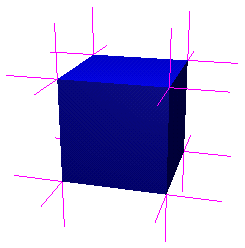#### 3. Cube with Unshared Vertices and Assigned Point Normals

The third cube is defined the same way as the second with unshared vertices, but the point normals have been defined in this example so that it will behave like the first cube with shared vertices when lit. The point of this example is to show the power of user assigned point normals.

```##################################################
# Cube with unshared vertices and point normals
#

point pNormalsXY ( 1  1  1)
normal ( 1  1  1)
endpoint
point pNormalsxY (-1  1  1)
normal (-1  1  1)
endpoint
point pNormalsXy ( 1 -1  1)
normal ( 1 -1  1)
endpoint
point pNormalsxy (-1 -1  1)
normal (-1 -1  1)
endpoint

face fNormals ( pNormalsXY pNormalsxY pNormalsxy pNormalsXy )
endface
```
```object oNormals ( fNormals )
endobject

group gCubeNormals
# Equivalent to fX
instance oNormals
rotate ( 0 1 0 ) ( 90 )
endinstance
# Equivalent to fx
instance oNormals
rotate ( 0 1 0 ) ( -90 )
endinstance
# Equivalent to fY
instance oNormals
rotate ( 1 0 0 ) ( -90 )
endinstance
# Equivalent to fy
instance oNormals
rotate ( 1 0 0 ) ( 90 )
endinstance
# Equivalent to fZ
instance oNormals
endinstance
# Equivalent to fz
instance oNormals
rotate ( 0 1 0 ) ( 180 )
endinstance
endgroup
```
Corner ZoomEach corner of this cube has 3 separate points, but all of the normals of these points have been assigned to be the vector along the space diagonal of the cube. The resulting images under lighting look exactly the same as the cube with shared vertices.### sphere

A sphere statement describes a portion of a spherical shell that is parameteratized based on latitude and longitude. The z-axis is the axis for the poles of the sphere.

SLIDE Definition tclinit Definition
 sphere id radius radius_float zmin zmin_float zmax zmax_float thetamax thetamax_float zslices zslices_int thetaslices thetaslices_int texture ( texture_triple ) [( texture_triple )] ( texture_triple ) [( texture_triple )] solid solid_flag surface surface_id shading shading_flag lod lod_flag ribbegin "rib_string" ribend "rib_string" endsphere
 slide create sphere id \ -radius radius_float \ -zmin zmin_float \ -zmax zmax_float \ -thetamax thetamax_float \ -zslices zslices_int \ -thetaslices thetaslices_int \ -texture { texture_triple } [{ texture_triple }] { texture_triple } [{ texture_triple }] \ -solid solid_flag \ -surface surface_id \ -shading shading_flag \ -lod lod_flag \ -ribbegin "rib_string" \ -ribend "rib_string" \

SLIDE Defaults tclinit Defaults
 sphere id radius `1.0` zmin `0.0` zmax `1.0` thetamax `360.0` zslices `8` thetaslices `8` texture ( `0.0` `0.0` ) [( `1.0` `0.0` )] ( `1.0` `1.0` ) [( `0.0` `1.0` )] solid `SLF_SOLID` surface `SLF_INHERIT` shading `SLF_INHERIT` lod `SLF_FULL` ribbegin `SLF_NULL` ribend `SLF_NULL` endsphere
 slide create sphere id \ -radius `1.0` \ -zmin `0.0` \ -zmax `1.0` \ -thetamax `360.0` \ -zslices `8` \ -thetaslices `8` \ -texture `{ 0.0 0.0 }` [`{ 1.0 0.0 }`] `{ 1.0 1.0 }` [`{ 0.0 1.0 }`] \ -solid `\$SLF_SOLID` \ -surface `\$SLF_INHERIT` \ -shading `\$SLF_INHERIT` \ -lod `\$SLF_FULL` \ -ribbegin `\$SLF_NULL` \ -ribend `\$SLF_NULL`Field Description

zmin

The minimum z value is expressed as a percentage of the diameter. This defines the minimum latitude line of the patch on the sphere. The surface is bounded by the plane parallel to the xy-plane at this z height. zmin can be greater than zmax in which case the surface will be turned inside out.

0.0 <= zmin <= 1.0

zmax

The maximum z value is expressed as a percentage of the diameter. This defines the maximum latitude line of the patch on the sphere. The surface is bounded by the plane parallel to the xy-plane at this z height. zmax can be less than zmax in which case the surface will be turned inside out.

0.0 <= zmax <= 1.0

thetamax

thetamax is specified in degrees. The surface begins at the x-axis and circles the z-axis by the angle theta until thetamax is reached.

zslices

The integer number of latitudinal triangle strips used to tesselate the sphere patch. The strips are separated evenly in angle.

0 < zslices

thetaslices

The integer number of longitudinal triangle strips used to tesselate the sphere patch. The strips are separated evenly in angle.

0 < thetaslices

### Example: Sphere PatchThis sphere patch was made using the primitives.slf example. The parameters below define the texture mapped patch, and the wire frame sphere illustrates the default parameter settings.

Static SLIDE Procedural SLIDE
```# Sphere Patch

sphere sph
surface sTile
solid SLF_HOLLOW
zmin 0.25
zmax 0.925
thetamax 67.0
texture ( 0 0 ) ( 1 1 )
endsphere
```
```# Sphere Patch
tclinit {
slide create sphere sph \
-surface sTile \
-solid SLF_HOLLOW \
-zmin 0.25 \
-zmax 0.925 \
-thetamax 67.0 \
-texture { 0 0 } { 1 1 }
}
```

### cylinder

A cylinder statement describes a portion of a cylindrical shell whose central axis is the z-axis.

SLIDE Definition tclinit Definition
 cylinder id radius radius_float zmin zmin_float zmax zmax_float thetamax thetamax_float zslices zslices_int thetaslices thetaslices_int begincap begincap_bool endcap endcap_bool texture ( texture_triple ) [( texture_triple )] ( texture_triple ) [( texture_triple )] solid solid_flag surface surface_id shading shading_flag lod lod_flag ribbegin "rib_string" ribend "rib_string" endcylinder
slide create cylinderid \
 -radius radius_float \ -zmin zmin_float \ -zmax zmax_float \ -thetamax thetamax_float \ -zslices zslices_int \ -thetaslices thetaslices_int \ -begincap begincap_bool -endcap endcap_bool -texture { texture_triple } [{ texture_triple }] { texture_triple } [{ texture_triple }] \ -solid solid_flag \ -surface surface_id \ -shading shading_flag \ -lod lod_flag \ -ribbegin "rib_string" \ -ribend "rib_string" \

SLIDE Defaults tclinit Defaults
 cylinder id radius `1.0` zmin `0.0` zmax `1.0` thetamax `360.0` zslices `1` thetaslices `8` begincap `0` endcap `0` texture ( `0.0` `0.0` ) [( `1.0` `0.0` )] ( `1.0` `1.0` ) [( `0.0` `1.0` )] solid `SLF_SOLID` surface `SLF_INHERIT` shading `SLF_INHERIT` lod `SLF_FULL` ribbegin `SLF_NULL` ribend `SLF_NULL` endcylinder
 slide create cylinder id \ -radius `1.0` \ -zmin `0.0` \ -zmax `1.0` \ -thetamax `360.0` \ -zslices `1` \ -thetaslices `8` \ -begincap `0` -endcap `0` -texture `{ 0.0 0.0 }` [`{ 1.0 0.0 }`] `{ 1.0 1.0 }` [`{ 0.0 1.0 }`] \ -solid `\$SLF_SOLID` \ -surface `\$SLF_INHERIT` \ -shading `\$SLF_INHERIT` \ -lod `\$SLF_FULL` \ -ribbegin `\$SLF_NULL` \ -ribend `\$SLF_NULL`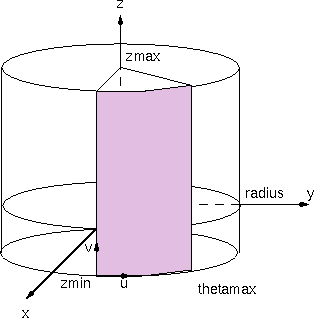Field Description

The radius of the circular cross section of the cylinder in the xy-plane.

zmin

The minimum z value, this defines the minimum latitude line of the patch on the cylinder. The surface is bounded by the plane parallel to the xy-plane at this z height. zmin can be greater than zmax in which case the surface will be turned inside out.

zmax

The maximum z value, this defines the maximum latitude line of the patch on the cylinder. The surface is bounded by the plane parallel to the xy-plane at this z height. zmax can be less than zmax in which case the surface will be turned inside out.

thetamax

thetamax is specified in degrees. The surface begins at the x-axis and circles the z-axis by the angle theta until thetamax is reached.

zslices

The integer number of triangle strips parallel to the xy-plane that are used to tesselate the cylinder patch. The strips are separated evenly in z height.

0 < zslices

thetaslices

The integer number of triangle strips parallel to the z-axis that are used to tesselate the cylinder patch. The strips are separated evenly in angle.

0 < thetaslices

begincap

Boolean that specifies whether or not to draw a cap at the zmin.

endcap

Boolean that specifies whether or not to draw a cap at the zmax.

### Example: Cylinder PatchThis cylinder patch was made using the primitives.slf example. The parameters below define the texture mapped patch, and the wire frame cylinder illustrates the default parameter settings.

Static SLIDE Procedural SLIDE
```# Cylinder Patch

cylinder cyl
surface sTile
solid SLF_HOLLOW
zmin -0.2
zmax 0.9
thetamax 67.0
texture ( 0 0 ) ( 1 1 )
endcylinder
```
```# Cylinder Patch
tclinit {
slide create cylinder cyl \
-surface sTile \
-solid SLF_HOLLOW \
-zmin -0.2 \
-zmax 0.9 \
-thetamax 67.0 \
-texture { 0 0 } { 1 1 }
}
```

### cone

A cone statement describes a portion of a cylindrical shell whose central axis is the z-axis.

SLIDE Definition tclinit Definition
 cone id radius radius_float height height_float zmin zmin_float zmax zmax_float thetamax thetamax_float zslices zslices_int thetaslices thetaslices_int begincap begincap_bool texture ( texture_triple ) [( texture_triple )] ( texture_triple ) [( texture_triple )] solid solid_flag surface surface_id shading shading_flag lod lod_flag ribbegin "rib_string" ribend "rib_string" endcone
 slide create cone id \ -radius radius_float \ -height height_float \ -zmin zmin_float \ -zmax zmax_float \ -thetamax thetamax_float \ -zslices zslices_int \ -thetaslices thetaslices_int \ -begincap begincap_bool -texture { texture_triple } [{ texture_triple }] { texture_triple } [{ texture_triple }] \ -solid solid_flag \ -surface surface_id \ -shading shading_flag \ -lod lod_flag \ -ribbegin "rib_string" \ -ribend "rib_string" \

SLIDE Defaults tclinit Defaults
 cone id radius `1.0` height `1.0` zmin `0.0` zmax `1.0` thetamax `360.0` zslices `1` thetaslices `8` begincap `0` texture ( `0.0` `0.0` ) [( `1.0` `0.0` )] ( `1.0` `1.0` ) [( `0.0` `1.0` )] solid `SLF_SOLID` surface `SLF_INHERIT` shading `SLF_INHERIT` lod `SLF_FULL` ribbegin `SLF_NULL` ribend `SLF_NULL` endcone
 slide create cone id \ -radius `1.0` \ -height `1.0` \ -zmin `0.0` \ -zmax `1.0` \ -thetamax `360.0` \ -zslices `1` \ -thetaslices `8` \ -begincap `0` -texture `{ 0.0 0.0 }` [`{ 1.0 0.0 }`] `{ 1.0 1.0 }` [`{ 0.0 1.0 }`] \ -solid `\$SLF_SOLID` \ -surface `\$SLF_INHERIT` \ -shading `\$SLF_INHERIT` \ -lod `\$SLF_FULL` \ -ribbegin `\$SLF_NULL` \ -ribend `\$SLF_NULL`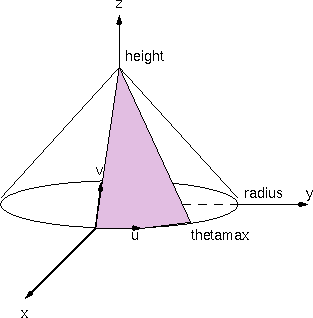Field Description

The radius of the circular base of the cone in the xy-plane.

height

The height value defines the position along the positive z-axis where the apex of the cone lies. If the height is zero then cone becomes a flat disk.

0.0 <= height

zmin

The minimum z value is expressed as a percentage of the height. This defines the minimum latitude line of the patch on the cone. The surface is bounded by the plane parallel to the xy-plane at this z height. zmin can be greater than zmax in which case the surface will be turned inside out.

0 <= zmin <= 1.0

zmax

The maximum z value is expressed as a percentage of the the height. This defines the maximum latitude line of the patch on the cone. The surface is bounded by the plane parallel to the xy-plane at this z height. zmax can be less than zmax in which case the surface will be turned inside out.

0 <= zmax <= 1.0

thetamax

thetamax is specified in degrees. The surface begins at the x-axis and circles the z-axis by the angle theta until thetamax is reached.

zslices

The integer number of concentric triangle strips that are used to tesselate the cone patch. The strips are separated evenly in z height.

0 < zslices

thetaslices

The integer number of radial triangle strips that are used to tesselate the cone patch. The strips are separated evenly in angle.

0 < thetaslices

begincap

Boolean that specifies whether or not to draw a cap at the zmin.

### Example: Cone PatchThis cone patch was made using the primitives.slf example. The parameters below define the texture mapped patch, and the wire frame cone illustrates the default parameter settings.

Static SLIDE Procedural SLIDE
```# Cone Patch

cone con
surface sTile
solid SLF_HOLLOW
height 0.0
zmin 0.0
zmax 1.0
thetamax 75.0
texture ( 0 0 ) ( 1 1 )
endcone
```
```# Cone Patch
tclinit {
slide create cone con \
-surface sTile \
-solid SLF_HOLLOW \
-height 0.0 \
-zmin 0.0 \
-zmax 1.0 \
-thetamax 75.0 \
-texture { 0 0 } { 1 1 }
}
```

### Example: DiskThis disk was made using cone primitive in the primitives.slf example. The parameters below define the texture mapped patch.

Static SLIDE Procedural SLIDE
```# Disk

cone conDisk
surface sTile
solid SLF_HOLLOW
height 0.0
zmin 0.0
zmax 1.0
thetamax 360.0
texture ( 0 0 ) ( 1 1 )
endcone
```
```# Disk
tclinit {
slide create cone conDisk \
-surface sTile \
-solid SLF_HOLLOW \
-height 0.0 \
-zmin 0.0 \
-zmax 1.0 \
-thetamax 360.0 \
-texture ( 0 0 ) ( 1 1 )
}
```

### Example: RingStatic SLIDE Procedural SLIDE
```# Ring

cone conRing
surface sTile
solid SLF_HOLLOW
height 0.0
zmin 0.0
zmax 0.75
thetamax 360.0
texture ( 0 0 ) ( 1 1 )
endcone
```
```# Ring
tclinit {
slide create cone conRing \
-surface sTile \
-solid SLF_HOLLOW \
-height 0.0 \
-zmin 0.0 \
-zmax 0.75 \
-thetamax 360.0 \
-texture ( 0 0 ) ( 1 1 )
}
```

### torus

A torus statement describes a portion of a toroidal shell whose major circular path lies in the xy-plane centered around the z-axis. The surface of the torus is constructed by sweeping the center of a circle with a radius equal to the minor radius along the path defined by the major circle.

SLIDE Definition tclinit Definition
 torus id majorradius majorradius_float minorradius minorradius_float thetamax thetamax_float phimin phimin_float phimax phimax_float phislices phislices_int thetaslices thetaslices_int texture ( texture_triple ) [( texture_triple )] ( texture_triple ) [( texture_triple )] solid solid_flag surface surface_id shading shading_flag lod lod_flag ribbegin "rib_string" ribend "rib_string" endtorus
 slide create torus id \ -majorradius majorradius_float \ -minorradius minorradius_float \ -thetamax thetamax_float \ -phimin phimin_float \ -phimax phimax_float \ -phislices phislices_int \ -thetaslices thetaslices_int \ -texture { texture_triple } [{ texture_triple }] { texture_triple } [{ texture_triple }] \ -solid solid_flag \ -surface surface_id \ -shading shading_flag \ -lod lod_flag \ -ribbegin "rib_string" \ -ribend "rib_string" \

SLIDE Defaults tclinit Defaults
 torus id majorradius `1.0` minorradius `0.5` thetamax `360.0` phimin `0.0` phimax `360.0` phislices `8` thetaslices `8` texture ( `0.0` `0.0` ) [( `1.0` `0.0` )] ( `1.0` `1.0` ) [( `0.0` `1.0` )] solid `SLF_SOLID` surface `SLF_INHERIT` shading `SLF_INHERIT` lod `SLF_FULL` ribbegin `SLF_NULL` ribend `SLF_NULL` endtorus
 slide create torus id \ -majorradius `1.0` \ -minorradius `0.5` \ -thetamax `360.0` \ -phimin `0.0` \ -phimax `360.0` \ -phislices `8` \ -thetaslices `8` \ -texture `{ 0.0 0.0 }` [`{ 1.0 0.0 }`] `{ 1.0 1.0 }` [`{ 0.0 1.0 }`] \ -solid `\$SLF_SOLID` \ -surface `\$SLF_INHERIT` \ -shading `\$SLF_INHERIT` \ -lod `\$SLF_FULL` \ -ribbegin `\$SLF_NULL` \ -ribend `\$SLF_NULL`Field Description

The radius of the circular axis curve of the torus around the z-axis in the xy-plane.

The radius of the circular cross section of the torus.

thetamax

thetamax is specified in degrees. The cross section circle is swept starting at the x-axis and circles the z-axis by the angle theta until thetamax is reached.

phimin

phimin is specified in degrees. When theta = 90, phi starts at the y-axis and rotates about the x-axis.phimin can be greater than phimax in which case the surface will be turned inside out.

phimax

phimax is specified in degrees. When theta = 90, phi starts at the y-axis and rotates about the x-axis.phimax can be less than phimin in which case the surface will be turned inside out.

thetaslices

The integer number of triangle strips parallel circling the circular axis that are used to tesselate the torus patch. The strips are separated evenly in theta angle.

0 < thetaslices

phislices

The integer number of triangle strips parallel to the circular axis that are used to tesselate the torus patch. The strips are separated evenly in phi angle.

0 < phislices

### Example: Torus PatchThis torus patch was made using the primitives.slf example. The parameters below define the texture mapped patch, and the wire frame torus illustrates the default parameter settings.

Static SLIDE Procedural SLIDE
```# Torus Patch

torus tor
surface sTile
solid SLF_HOLLOW
thetamax 90.0
phimin 0.0
phimax 90.0
texture ( 0 0 ) ( 1 1 )
endtorus
```
```# Torus Patch
tclinit {
slide create torus tor \
-surface sTile \
-solid SLF_HOLLOW \
-thetamax 90.0 \
-phimin 0.0 \
-phimax 90.0 \
-texture { 0 0 } { 1 1 }
}
```

### cyclide

SLIDE Definition tclinit Definition
 cyclide id drawellipse drawellipse_bool drawhyperbola drawhyperbola_bool majorradius majorradius_float minorradius minorradius_float stringlength stringlength_float thetamin thetamin_float thetamax thetamax_float phimin phimin_float phimax phimax_float phislices phislices_int thetaslices thetaslices_int texture ( texture_triple ) [( texture_triple )] ( texture_triple ) [( texture_triple )] solid solid_flag surface surface_id shading shading_flag lod lod_flag ribbegin "rib_string" ribend "rib_string" endcyclide
 slide create cyclide id \ -drawellipse drawellipse_bool \ -drawhyperbola drawhyperbola_bool \ -majorradius majorradius_float \ -minorradius minorradius_float \ -stringlength stringlength_float \ -thetamin thetamin_float \ -thetamax thetamax_float \ -phimin phimin_float \ -phimax phimax_float \ -phislices phislices_int \ -thetaslices thetaslices_int \ -texture { texture_triple } [{ texture_triple }] { texture_triple } [{ texture_triple }] \ -solid solid_flag \ -surface surface_id \ -shading shading_flag \ -lod lod_flag \ -ribbegin "rib_string" \ -ribend "rib_string" \
SLIDE Defaults tclinit Defaults
 cyclide id drawellipse 0 drawhyperbola 0 majorradius 0.5 minoradius 0.4 stringlength 0.8 thetamin 0.0 thetamax 360.0 phimin 0.0 phimax 360.0 phislices 8 thetaslices 8 texture ( 0.0 0.0 ) [( 1.0 0.0 )] ( 1.0 1.0 ) [( 0.0 1.0 )]  solid SLF_SOLID surface SLF_INHERIT shading SLF_INHERIT lod SLF_FULL ribbegin SLF_NULL ribend SLF_NULL endcyclide
 slide create cyclide id \ -drawellipse `0` \ -drawhyperbola`0` \ -majorradius `0.5` \ -minorradius `0.4` \ -stringlength `0.8` \ -thetamin `0.0` \ -thetamax `360.0` \ -phimin `0.0` \ -phimax `360.0` \ -phislices `8` \ -thetaslices `8` \ -texture `{ 0.0 0.0 }` [`{ 1.0 0.0 }`] `{ 1.0 1.0 }` [`{ 0.0 1.0 }`] \ -solid `\$SLF_SOLID` \ -surface `\$SLF_INHERIT` \ -shading `\$SLF_INHERIT` \ -lod `\$SLF_FULL` \ -ribbegin `\$SLF_NULL` \ -ribend `\$SLF_NULL`A cyclide statement describes a portion of a cyclide shell. A cyclide is constructed with an ellipse and a hyperbola that is confocal to the ellipse. One end of a string, with length stringlength_float >= 0, is attached to the -x focus of the ellipse. The string is pulled taught to a point on the ellipse and then the remaining length of the string is swept around the ellipse by tracing its focal ray through every point on the hyperbola.

The ellipse is centered at the origin with majorradius in the x-axis, and minorradius in the y-axis, such that 0 < minorradius <= majorradius. The string is pulled taught around the ellipse between angle thetamin_float to angle thetamax_float from the x-axis. The string is swept around the ellipse between angle phimin_float to angle phimax_float.

0 <= phimin, phimax, thetamin, thetamax <= 720.
If thetamin_float > thetamax_float or it phimin_float > phimax_float, then the orientation of the shell is reversed.

The integer parameters, phislices_int and thetaslices_int describe the fineness of the tesselation to be used when rendering the torus with polygons; phislices_int, thetaslices_int >= 0 .